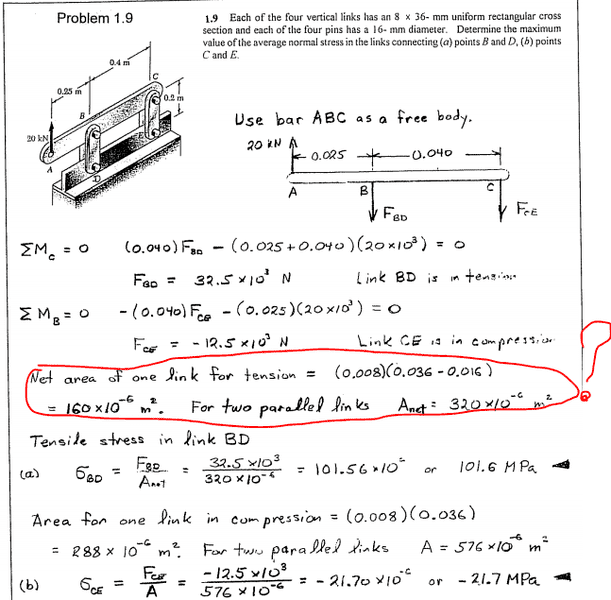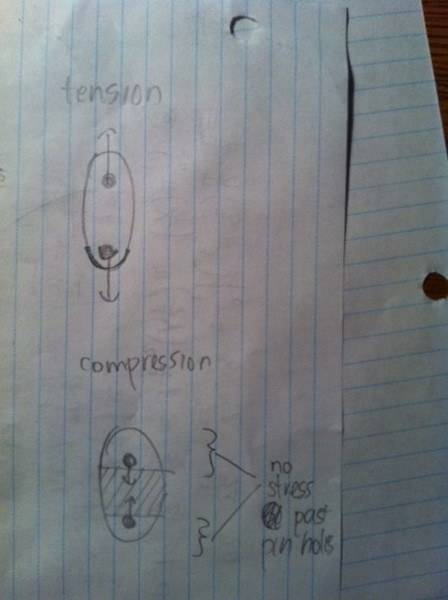# Finding the max value of the average normal stress

theBEAST

## Homework Statement

Here is the question with the solution:## The Attempt at a Solution

I understand the first part where they find the forces by summation of moments. However I don't understand how they got that area. Why would they take the diameter (0.016) and subtract it from the length (0.036)? Aren't you suppose to use the area of the link that is parallel to the force. In other words, use the area (0.008) * (the thickness of the link which is not given)?

For example if you were pulling a cylindrical rod you would use the area of the circle to find the stress and not the surface area of the sides of the cylinder.

Homework Helper
Gold Member
The thickness is given. The link has a horizontal cross section of 8 x 36mm stated in the first line of the problem. As for the area calculated...think about where on the link the max stress occurs.

theBEAST
The thickness is given. The link has a horizontal cross section of 8 x 36mm stated in the first line of the problem. As for the area calculated...think about where on the link the max stress occurs.

Oh I see, but why is it that the link in tension has an area 0.008 * (0.036-0.016) whereas the link in compression is just 0.008 * 0.036?

Homework Helper
Gold Member
Oh I see, but why is it that the link in tension has an area 0.008 * (0.036-0.016) whereas the link in compression is just 0.008 * 0.036?
When in compression, are there any stresses in the links at the ends beyond the pin holes?

theBEAST
When in compression, are there any stresses in the links at the ends beyond the pin holes?

Hmm... Well how I see it is that the max stress is applied at where the pin holes are. In other words I think the area for both should be 0.008 * 0.016 (the width of the link times the diameter of the whole). What I see is that we are just pulling the pins... Sorry I am just very confused, could you explain why my hypothesis is incorrect?

Homework Helper
Gold Member
There is a difference between normal tensile or compressive stresses and bearing stresses. When you calculate the area based on thickness times hole diameter, you are calculating the area to use for localized bearing stresses. These stresses exist at the hole and pin regardless of wheteher loading in the link is tensile or compressive. Allowable bearing (crushing) stresses are quite high compared to allowable normal stresses in the link itself. The hole decreases the net area of the link cross section when the member is in tension. But when in compression...??

theBEAST
There is a difference between normal tensile or compressive stresses and bearing stresses. When you calculate the area based on thickness times hole diameter, you are calculating the area to use for localized bearing stresses. These stresses exist at the hole and pin regardless of wheteher loading in the link is tensile or compressive. Allowable bearing (crushing) stresses are quite high compared to allowable normal stresses in the link itself. The hole decreases the net area of the link cross section when the member is in tension. But when in compression...??

WOW I think I am starting to understand this :D Alright I understand why we use that area for compression as I tried to illustrate in this diagram. Since the compression stress pushes inwards, then we must use the entire cross sectional area.However I am still a bit lost with tension, if you look at how I bolded the bottom part of the link in tension it still looks to me as if the whole area 0.008*0.036 is under tension. Could you please explain how I should be visualizing this?

Thanks!

Homework Helper
Gold Member
WOW I think I am starting to understand this :D Alright I understand why we use that area for compression as I tried to illustrate in this diagram. Since the compression stress pushes inwards, then we must use the entire cross sectional area.However I am still a bit lost with tension, if you look at how I bolded the bottom part of the link in tension it still looks to me as if the whole area 0.008*0.036 is under tension. Could you please explain how I should be visualizing this?

Thanks!
For the compression case, you cross - hatched the area of the compressive stresses, the area between the inside faces of the holes.
For the tension case, in a similar manner, cross hatch the area where the tensile stesses exist; but this time, that will be the area between the outside faces of the holes.
Tensile and compressive forces act perpendicular (normal) to the plane on which they act.